SSC BOARD PAPERS IMPORTANT TOPICS COVERED FOR BOARD EXAM 2024

### MENSURATION FORMULA

1. Area of rectangle (A) = length(l) * Breath(b)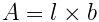2. Perimeter of a rectangle (P) = 2 * (Length(l) + Breath(b))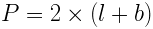3. Area of a square (A) = Length (l) * Length (l)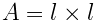4. Perimeter of a square (P) = 4 * Length (l)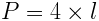5. Area of a parallelogram(A) = Length(l) * Height(h)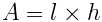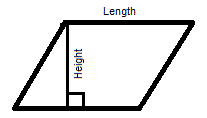6. Perimeter of a parallelogram (P) = 2 * (length(l) + Breadth(b))7. Area of a triangle (A) = (Base(b) * Height(b)) / 2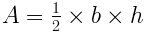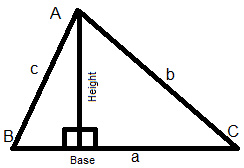And for a triangle with sides measuring “a” , “b” and “c” , Perimeter = a+b+c

and s = semi perimeter = perimeter / 2 = (a+b+c)/2

And also . Area of triangle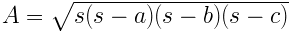This formulas is also knows as “Heroe’s formula”.

8. Area of triangle(A) =

Where , A , B and C are the vertex and angle A , B , C are respective angles of triangles and  a , b , c are the respective opposite sides of the angles as shown in figure below: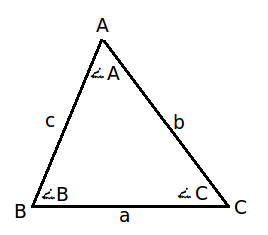area of triangle - mensuration

9. Area of isosceles triangle =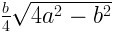Where , a = length of two equal side , b= length of base of isosceles triangle.

10. Area of trapezium (A) =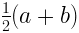Where , “a” and “b” are the length of parallel sides.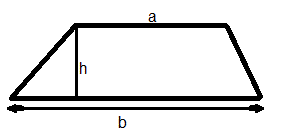11. Perimeter of a trapezium (P) = sum of all sides

12. Area f rhombus (A) =  Product of diagonals / 2

13. Perimeter of a rhombus (P) = 4 * l
where l = length of a side

14. Area of quadrilateral (A) = 1/2 * Diagonal * (Sum of offsets)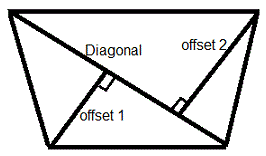15.  Area of a Kite (A) = 1/2 * product of it’s diagonals

16. Perimeter of a Kite (A) = 2 * Sum on non-adjacent sides

17.  Area of a Circle (A) =Where , r= radius of the circle and d= diameter of the circle.

18. Circumference of a Circle =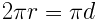d= diameter of circle

19. Total surface area of cuboid =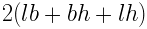where , l= length , b=breadth , h=height

20. Total surface area of cuboid =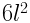where , l= length

21. length of diagonal of cuboid =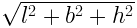22. length of diagonal of cube =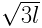23. Volume of cuboid = l * b * h

24. Volume of cube = l * l* l

25. Area of base of a cone =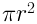26.  Curved surface area of a cone = C
Where , r = radius of base , l = slanting height of cone

27. Total surface area of a cone =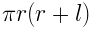28. Volume of right circular cone =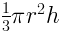Where , r = radius of base of cone , h= height of the cone (perpendicular to base)

29. Surface area of triangular prism = (P * height) + (2 * area of triangle)
Where , p = perimeter of base

30. Surface area of polygonal prism = (Perimeter of base * height ) + (Area of polygonal base * 2)

31. Lateral surface area of prism = Perimeter of base * height

32. Volume of  Triangular prism = Area of the triangular base * height

33. Curved surface area of  a cylinder =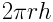Where , r = radius of base , h = height of cylinder

34. Total surface area of a cylinder =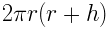35. Volume of a cylinder =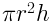36. Surface area of sphere =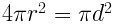where , r= radius of sphere , d= diameter of sphere

37. Volume of a sphere =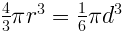38. Volume of hollow cylinder =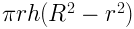where , R = radius of cylinder , r= radius of hollow , h = height of cylinder

39. Surface area of a right square pyramid =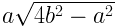Where , a = length of base , b= length of equal side  ; of the isosceles triangle forming the slanting face.

40. Volume of a right square pyramid =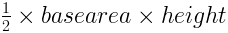41. Area of a regular hexagon =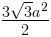42. area of equilateral triangle =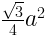43. Curved surface area of a Frustums =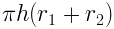44. Total surface area of a Frustums =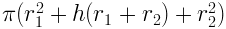45. Curved surface area of a Hemisphere =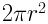46. Total surface area of a Hemisphere =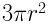47. Volume of a Hemisphere =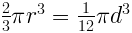48. Area of sector of a circle =where ,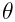= measure of angle of the sector , r= radius of the sector